May 26, 2023, 7:11 a.m.

This game is an economic strategy. It is the game where you can manage, optimize, distribute passenger traffic and build your own successful airline. The game is made in a minimalist style and is not complicated by unnecessary elements such as:
- emission into the environment;
- number of seats in business class;
- managing schedule; etc.

In this article, I will tell you about the main game mechanic without which there would not have been this game. It is the passenger traffic system.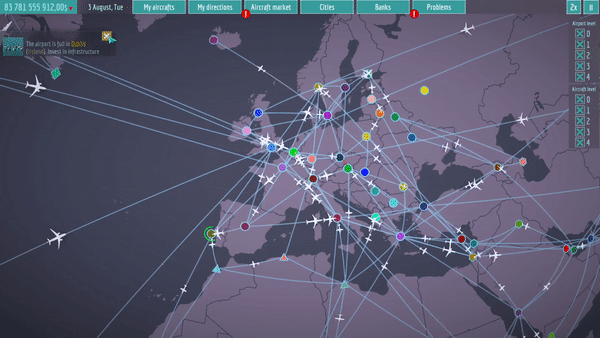The first thing, I would like to find the shortest way to a destination. I faced the problem of searching solution. I was starting to find the plugins for this. But all wasn`t easy because the game world is the sphere.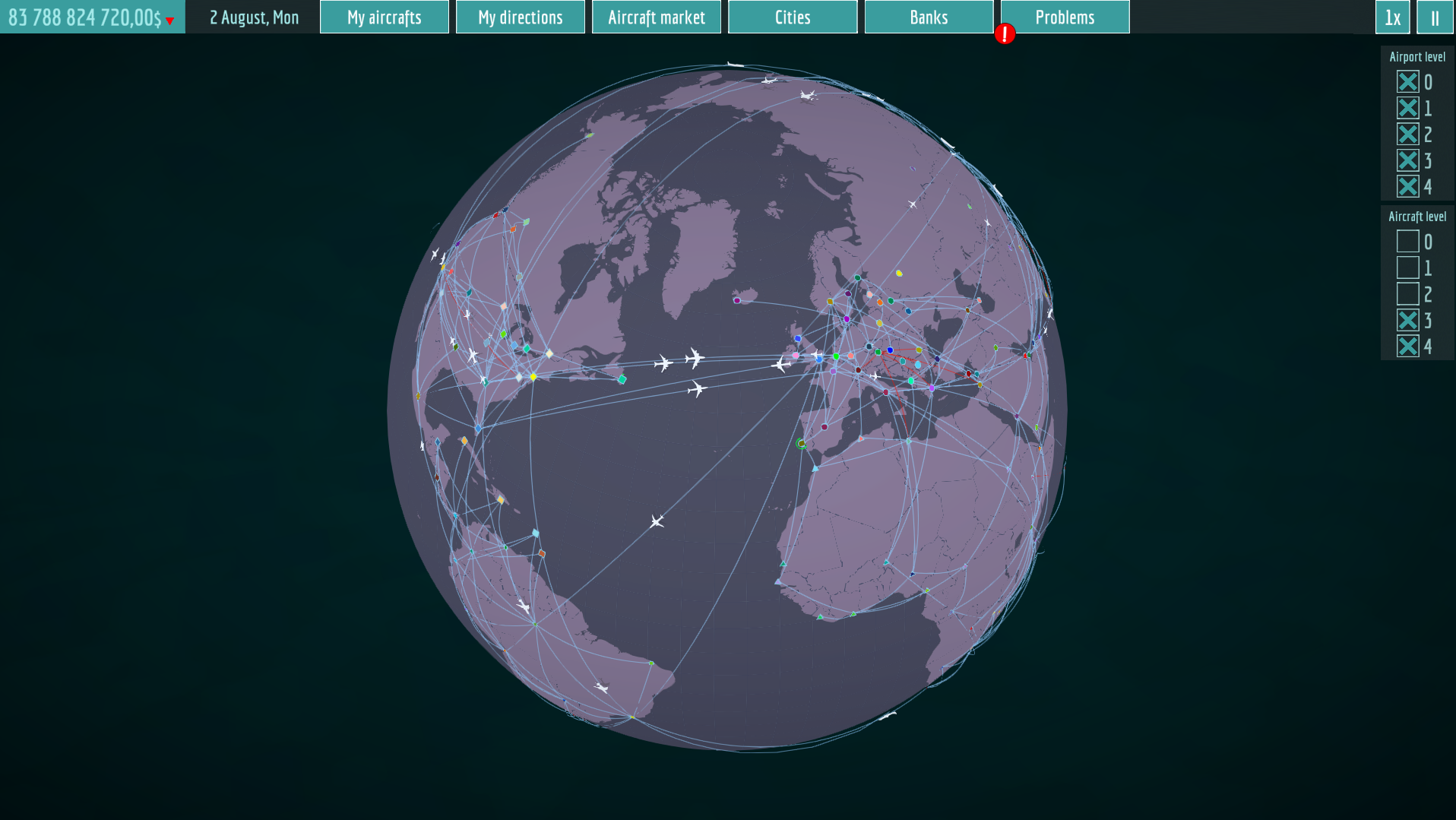As an option to solve this problem, I could create all routes in advance. Since there are 144 cities in the game and a player can create routes in any order the number of potential routes would be 20736. This could lead to indeterminacy and chaos in movement of passengers.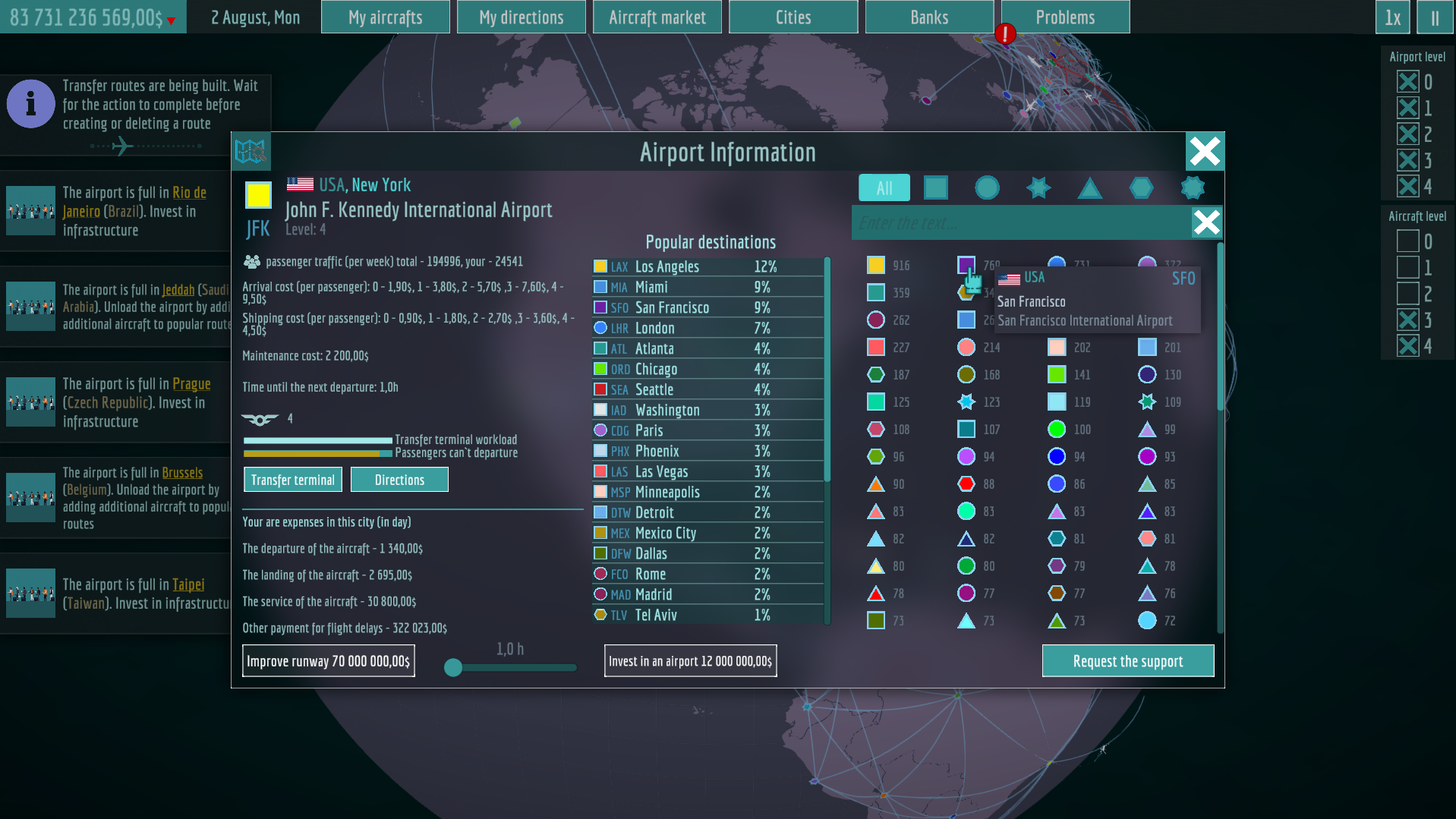So, I had to look for the shortest way after a player creates a route.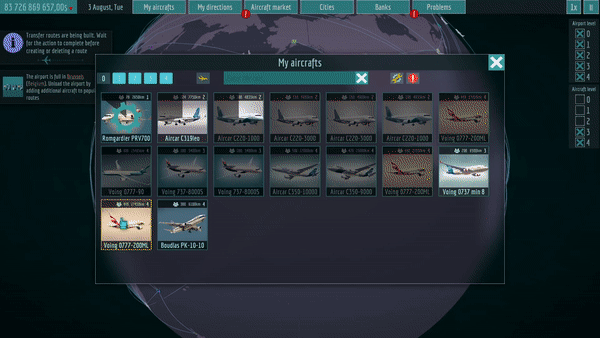I used Floyd-Warshall`s algorithm for solving this task. The algorithm works step by step. At each step, it goes through all vertices and checks the ability to pass from one to another through the current vertex. The algorithm is executed until all possible routes are found . So we will get the matrix of the distances among all pairs of vertices.

For example: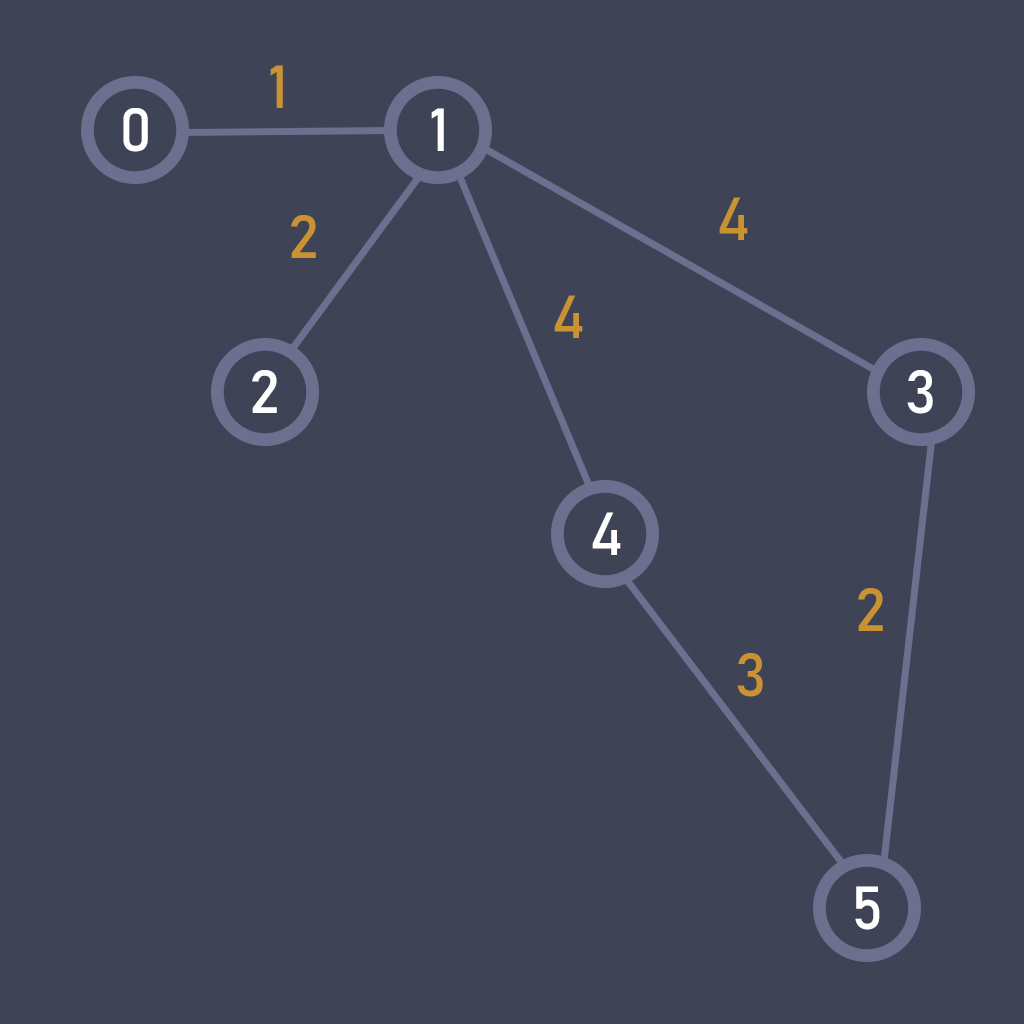We have points and distances among them. To find out the shortest way, we have to create a matrix of distances. The game is developed by Unity Engine so I will use C#.

We have to create a two-dimensional array where 999 is infinity when points aren`t connected with each other and other digits are the distances between the connected points.

int[,] graph = new int[6, 6]
{
{ 999, 1, 999, 999, 999, 999},
{ 1, 999, 2, 4, 4, 999},
{ 999, 2, 999, 999, 999, 999},
{ 999, 4, 999, 999, 2, 999},
{ 999, 4, 999, 2, 999, 3},
{ 999, 999, 999, 999, 3, 999},
};

The search algorithm itself looks like this.

int[,] distance = new int[graph.GetLength(0), graph.GetLength(1)];
int[,] finishMatrix = new int[graph.GetLength(0), graph.GetLength(1)];

for (int i = 0; i < graph.GetLength(0); ++i)
{
for (int j = 0; j < graph.GetLength(1); ++j)
{
distance[i , j] = graph[i , j];
finishMatrix[i , j] = j;
}
}

for (int k = 0; k < graph.GetLength(0); ++k)
{
for (int i = 0; i < graph.GetLength(0); ++i)
{
if (distance[i , k] != 999)
{
for (int j = 0; j < graph.GetLength(1); ++j)
{
if (distance[i , j] > distance[i , k] + distance[k , j])
{
distance[i , j] = distance[i , k] + distance[k , j];
finishMatrix[i , j] = finishMatrix[i , k];
}
}
}
}
}

This is the working example but we remember that in my game can be 20736 routes and this is too slow for this quantity. There for, there are various optimizations so that the miscalculation doesn`t affect the player`s experience in the game.

Finally, we launch it and see the result.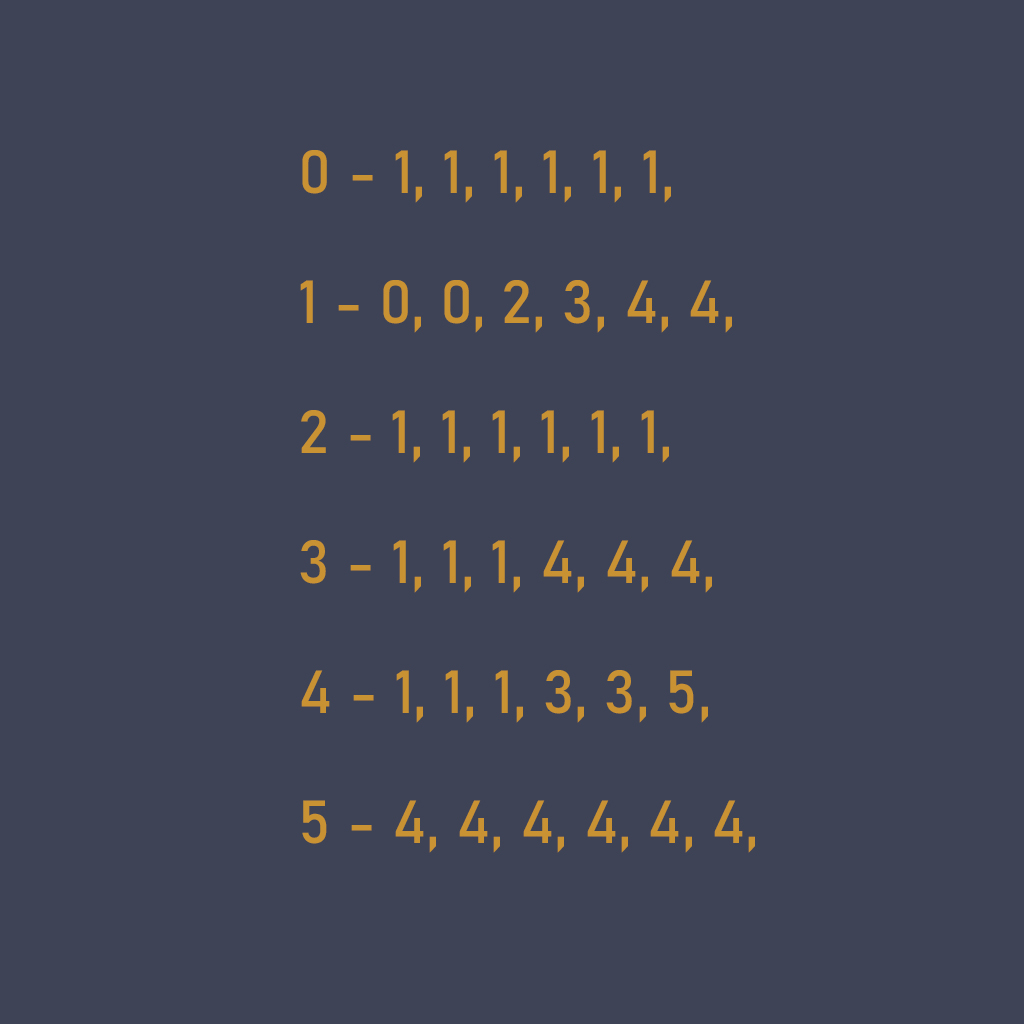We have got the final matrix and at the first glance we can `t see the way itself.
The next step is taking the finish point for example 3. 3 is the column 0 is the row and the start point.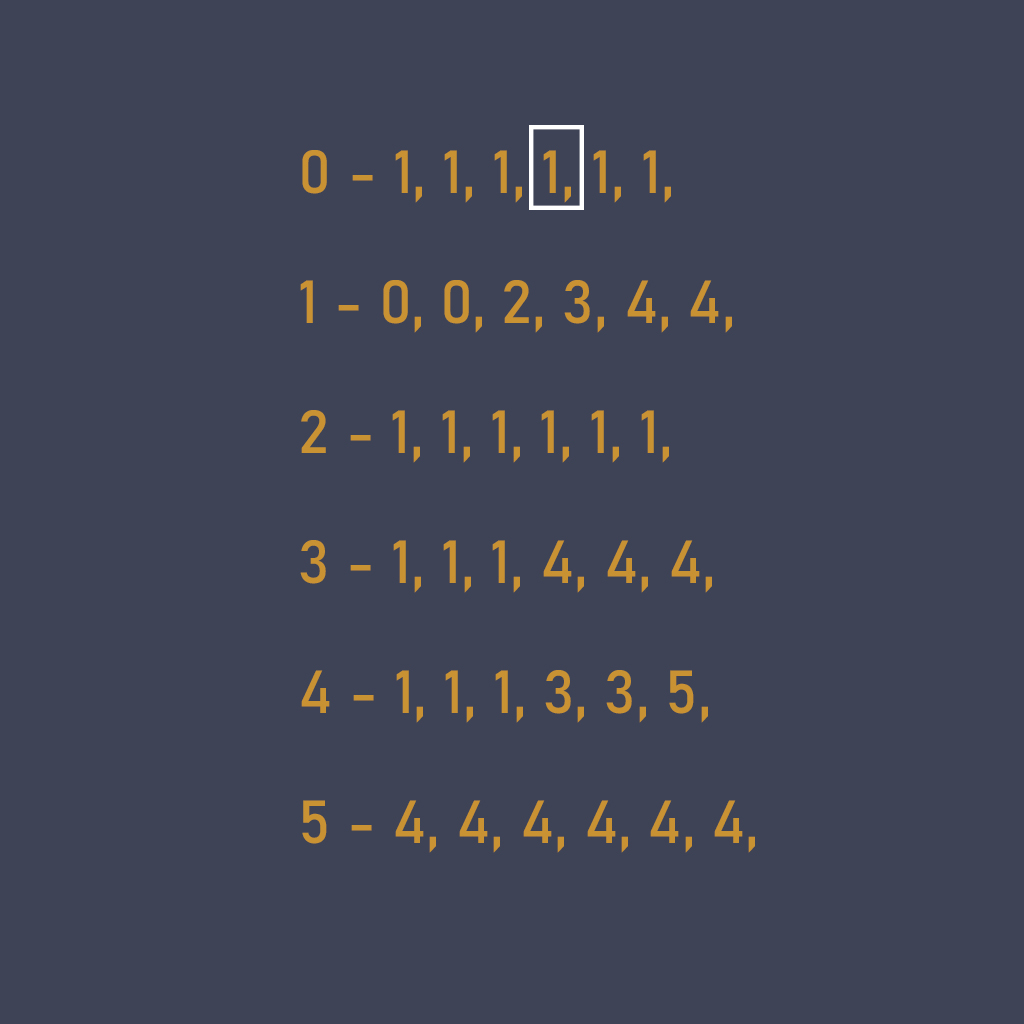You can see, we have found out from 0 to 3 but this way is quite easy.

Let`s take the finish point 5. There are two ways but it is easy to 0,1,4,5 is the best. This is easy for a human. But how can the program understand it?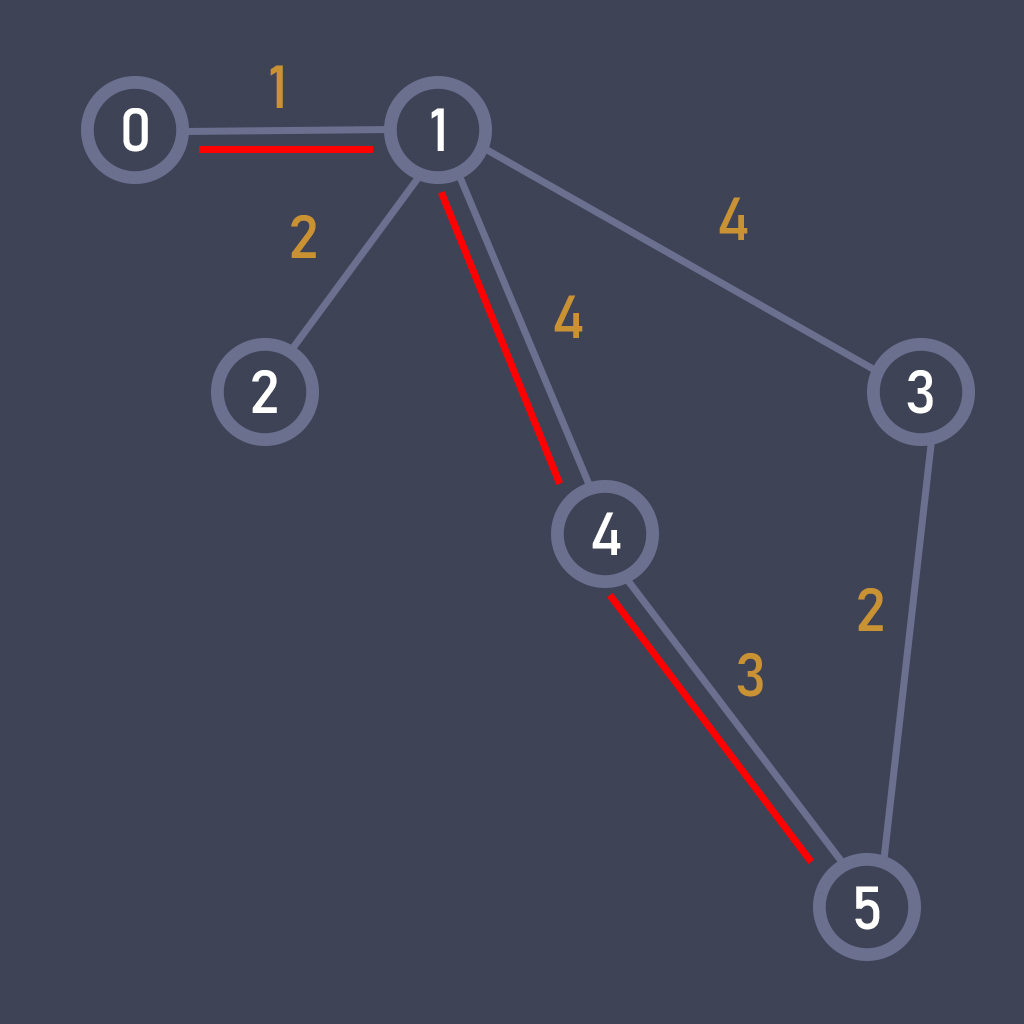Greate, we have found out the way through out our pre-prepared matrix. As, I wrote above the option of calculating routes isn`t suitable in advance, so I have wrote my rather complex algorithm for a creating distance matrix. By the way you can search for paths from different points yourself, start and finish points can be any.

Now the passengers can take the destination point even if there is no direction route.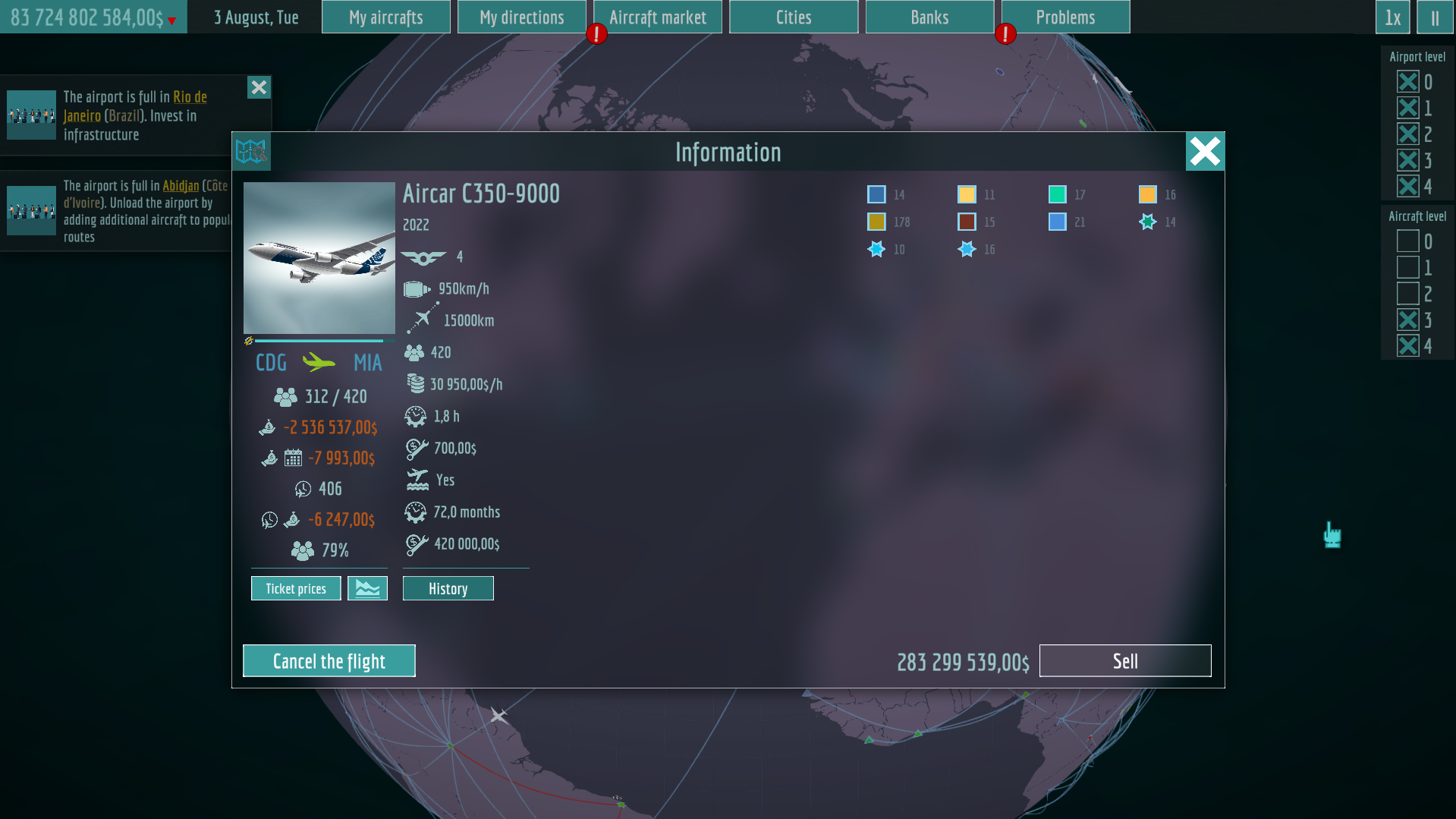The game will be released on  9 June 2023 on Steam, Nintendo Switch, IOS, Android

https://store.steampowered.com/app/2289080/Avia_corporation/